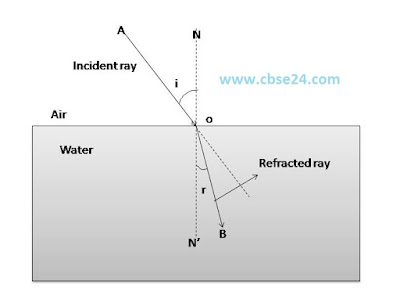# Laws of Refraction of light class 10 | cbse24

The phenomenon of refraction of light obeys the following two laws.

1:First law :-The incident ray, the refracted ray and the normal to the interface at the point of incident all lie in the some plane.Example:-AO(incident ray ) OB(Refracted ray) and normal NN' all lie in the same plane(which is the plane of the paper)

2:Second law:
The ratio of sin of the angle of incidence and the sin of the angle of refraction is constant for a given pair of media.

Where μ is refractive index of second medium with respect of first medium

### Refraction index in terms of speed of light:-

Refractive index of a medium with respect to vacuum is also called absolute refractive index

### Factor on which the refractive index of a medium depends

1:Nature of the medium

2:Wavelength of the medium

3:Temperature

4:Nature of the surrounding medium

Note:
•    mean refractive index of medium 2 with respect to medium 1
•   mean refractive index of medium 1 with respect to medium2
• A substance having higher refractive index is optically denser than another substance having lower refractive index.μ↑ than density ↑

*